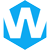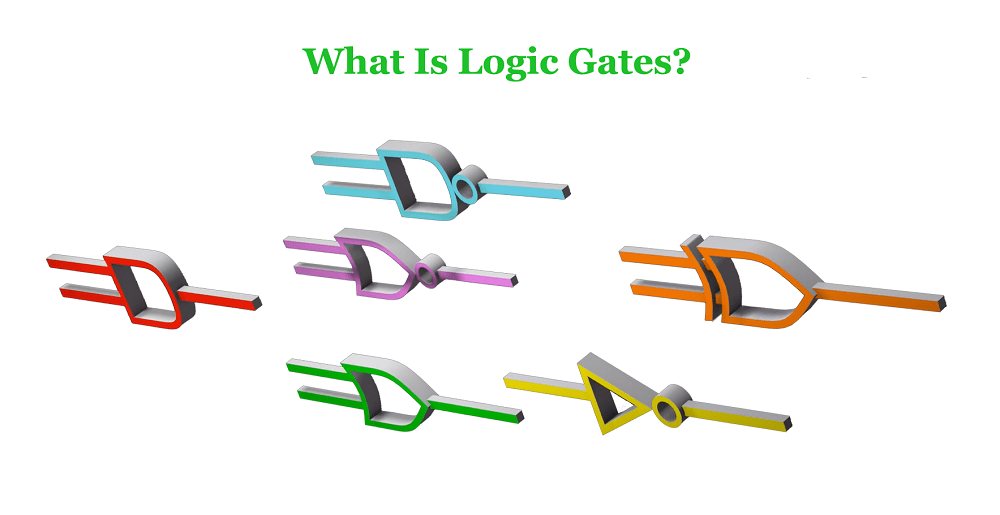# Logic Gates: Tyles, Symbol, Truth TableLogic Gates are the basic components on Integrated Circuits. Simple logic gates can be made of transistors. The combination of these transistors can make the high and low levels representing the two signals pass through them to generate a high or low level signal. High and low levels can respectively represent logical “true” and “false” or 1 and 0 in binary, thereby realizing logic operations.

## Basic Logic Gates

Here we will introduce 7 basic logic gates: AND gate, OR gate, NOT gate, NAND gate, NOR gate, XOR gate and XNOR gate. You‘ll learn their logical symbols, logical relations and truth tables.

### AND Gate

AND gate is also called “AND circuit”, logic “AND” circuit. It is a basic logic gate circuit that performs “AND” operation. There are multiple inputs and one output.

The output is high when all inputs are high (logic 1) at the same time, otherwise the output is low (logic 0).

The mathematical logic expression of AND gate: Y = AB, the truth table is as follows:AND Gate Truth Table
InputOutput
ABY
000
010
100
111

### OR Gate

OR gate, also known as OR circuit, logic and circuit. If one of several conditions is met, an event will occur. This relationship is called an “or” logical relationship. A circuit with an “OR” logic relationship is called an OR gate.

The OR gate has multiple inputs and one output, as long as one of the inputs is high (logic “1”), the output is high (logic “1”). The output is low (logic “0”) only when all inputs are low (logic “0”).

The mathematical logic expression of the OR gate is: Y=A+B=(A’B’)’, and its logical symbols and truth table are as follows:OR Gate Truth Table
InputOutput
ABY
000
011
101
111

### NOT Gate

A NOT gate, also known as a NOT circuit, an inverter, or a logic negation circuit, is the basic unit of a logic circuit. A NOT gate has one input and one output.

When the input is high (logic “1”), the output is low (logic “0”); conversely, when the input is low (logic “0”), the output is high level (logic “1”). The logic function of the NOT gate is equivalent to the NOT in the logic algebra, and the circuit function is equivalent to the inversion. This operation is also called the NOT operation.

The mathematical logic expression of the NOT gate is: F=A , and its logical symbols and truth table are as follows:NOT Gate Truth Table
InputOutput
AY
01
10

### NAND Gate

NAND gate is a basic logic circuit of digital circuit. It is a superposition of AND and NOT gates, with multiple inputs and one output.

The AND operation is performed first, followed by the NOT operation. When both inputs are high (1), the output is low (0); if at least one of the inputs is low (0), the output is high (1).

The logic symbol and truth table of the NAND gate are as follows:NAND Gate Truth Table
InputOutput
ABY
001
011
101
110

### NOR Gate

The NOR gate is the basic element in the digital logic circuit, which realizes the logical OR function. It has multiple inputs, 1 output, and the multi-input NOR gate can be composed of 2-input NOR gate and inverter.

The output is high (logic 1) when both inputs A and B are low (logic 0). When any input is high (logic 1), the output is low (logic 0).

The mathematical expression of its logical relationship is: Y=A+B, the logical symbol and truth table of the NOR gate are shown in the table below.NOR Gate Truth Table
InputOutput
ABY
001
010
100
110

### XOR Gate

An XOR gate is a logic gate that implements a logical XOR in digital logic. There are multiple inputs and one output, and the multi-input XOR gate can be composed of two-input XOR gates.

If the levels of the two inputs are different, the output is a high level 1; if the levels of the two inputs are the same, the output is a low level 0. That is, if the two inputs are different, the XOR gate outputs a high level 1.

The logical expression of the XOR gate: Y=A⊕B=A•B’+A’•B, the logical symbol and truth table of the XOR gate are as follows:XOR Gate Truth Table
InputOutput
ABY
000
011
101
110

### XNOR Gate

XNOR gate, also called equivalence gate, is the basic unit of digital logic circuit. It is formed by adding a NOT gate to the output of the XOR gate, and has 2 input terminals and 1 output terminal.

When one and only one of the two inputs is low (logic 0), the output is low. When the levels at the 2 inputs are the same, the output is high (logic 1).

Logical expression: F=A⊙B=A•B+A’•B’=(AB)’, the logic symbol and truth table of XNOR gate are as follows:XNOR Gate Truth Table
InputOutput
ABY
001
010
100
111

#### Share:Aidan Taylor

I am Aidan Taylor and I have over 10 years of experience in the field of PCB Reverse Engineering, PCB design and IC Unlock.

## Recommended Post

### Need Help?

Don't hesitate - Get in touch today to find out how we can help take your project to the next level.

Scroll to Top## Start to Achieve Your PCB Project

Hello 👋
Can we help you? Chat with us on WhatsApp↓, or send an email to us: info@reversepcb.com